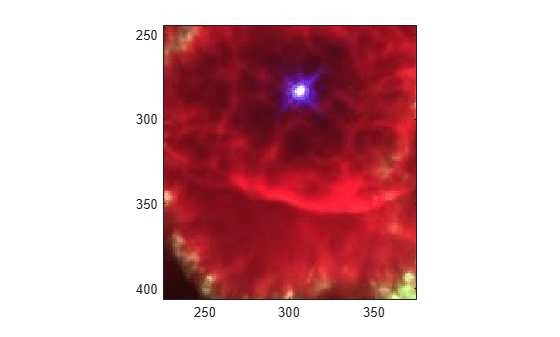rgb2ind

Convert RGB image to indexed image

Description

example

[X,cmap] = rgb2ind(RGB,Q) converts the RGB image to an indexed image X with associated colormap cmap using minimum variance quantization with Q quantized colors and dithering.

[X,cmap] = rgb2ind(RGB,tol) converts the RGB image to an indexed image using uniform quantization with tolerance tol and dithering.

X = rgb2ind(RGB,inmap) converts the RGB image to an indexed image using the inverse colormap algorithm with specified colormap inmap and dithering.

___ = rgb2ind(___,dithering) enables or disables dithering.

Examples

collapse all

Read and display a truecolor uint8 JPEG image of a nebula.

figure
imagesc(RGB)
axis image
zoom(4)Convert RGB to an indexed image with 32 colors.

[IND,map] = rgb2ind(RGB,32);
figure
imagesc(IND)
colormap(map)
axis image
zoom(4)Input Arguments

collapse all

RGB image, specified as an m-by-n-by-3 array.

Data Types: single | double | uint8 | uint16

Number of quantized colors used for minimum variance quantization, specified as a positive integer that is less than or equal to 65,536. The returned colormap cmap has Q or fewer colors.

Tolerance used for uniform quantization, specified as a number in the range [0, 1]. The returned colormap cmap has (floor(1/tol)+1)^3 or fewer colors.

Input colormap, specified as a c-by-3 matrix with values in the range [0, 1]. Each row of inmap is a three-element RGB triplet that specifies the red, green, and blue components of a single color of the colormap. The colormap has a maximum of 65,536 colors.

Data Types: double

Perform dithering, specified as 'dither' or 'nodither'. Dithering increases the color resolution at the expense of spatial resolution. For more information, see dither.

If you select 'nodither', then rgb2ind does not perform dithering. Instead, the function maps each color in the original image to the closest color in the new colormap.

Output Arguments

collapse all

Indexed image, returned as an m-by-n matrix of nonnegative integers. If the length of map is less than or equal to 256, then the output image is of class uint8. Otherwise, the output image is of class uint16. The value 0 in the output array X corresponds to the first color in the colormap.

Note

The values in image X are indexes into the colormap map and should not be used in mathematical processing, such as filtering operations.

Data Types: uint8 | uint16

Colormap, returned as a c-by-3 matrix with values in the range [0, 1]. Each row of cmap is a three-element RGB triplet that specifies the red, green, and blue components of a single color of the colormap. The colormap has a maximum of 65,536 colors.

Data Types: double

Algorithms

• Uniform Quantization — If you specify tol, then rgb2ind uses uniform quantization to convert the image. Uniform quantization cuts the RGB color cube into smaller cubes of length tol. For example, if you specify a tol of 0.1, then the edges of the cubes are one-tenth the length of the RGB cube. The total number of small cubes is:

t = (floor(1/tol)+1)^3

Each cube represents a single color in the output image. Therefore, t is the maximum length of the colormap . rgb2ind removes any colors that don’t appear in the input image, so the actual colormap can be smaller than t.

• Minimum Variance Quantization — If you specify Q, then rgb2ind uses minimum variance quantization. Minimum variance quantization cuts the RGB color cube into smaller boxes (not necessarily cubes) of different sizes, depending on how the colors are distributed in the image. If the input image actually uses fewer colors than the number specified, then the output colormap is also smaller.

• Inverse Colormap — If you specify an input colormap inmap, then rgb2ind uses colormap mapping. The inverse colormap algorithm quantizes the specified colormap into 32 distinct levels per color component. Then, for each pixel in the input image, the closest color in the quantized colormap is found.

 Spencer W. Thomas, "Efficient Inverse Color Map Computation", Graphics Gems II, (ed. James Arvo), Academic Press: Boston. 1991. (includes source code)